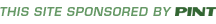home » reference » appendix b » measurements

# CSS Measurements

CSS supports a number of measurements including absolute units such as inches, centimeters, points, and so on, as well as relative measures such as percentages and em units. Table B-2 summarizes these units of measure.

 UnitDescriptionExample% Defines a measurement as a percentage relative to another value, typically an enclosing element. p {font-size: 14pt; line-height: 150%;} cm Defines a measurement in centimeters. div {margin-bottom: 1cm;} em A relative measurement for the height of a font in em spaces. Because an em unit is equivalent to the size of a given font, if you assign a font to 12pt, each "em" unit would be 12pt; thus, 2em would be 24pt. p {letter-spacing: 5em;} ex This value defines a measurement relative to a font's x-height. The x-height is determined by the height of the font's lowercase letter x. p {font-size: 14pt; line-height: 2ex;} in Defines a measurement in inches. p {word-spacing: .25in;} mm Defines a measurement in millimeters. p {word-spacing: 12mm;} pc Defines a measurement in picas. A pica is equivalent to 12 points; thus, there are 6 picas per inch. p {font-size: 10pc;} pt Defines a measurement in points. A point is defined as 1/72nd of an inch. body {font-size: 14pt;} px Defines a measurement in screen pixels. p {padding: 15px;} table b-2(X)HTML ElementsCSS PropertiesBack: Inclusion Next: Color Values< Home | About | Chapters | Examples | Errata | Reference | Site Map > © 1997-2005 PINT Inc. [contents and programming of the site] © 2003 The McGraw-Hill Companies [contents of the print work HTML/XHTML: The Complete Reference]